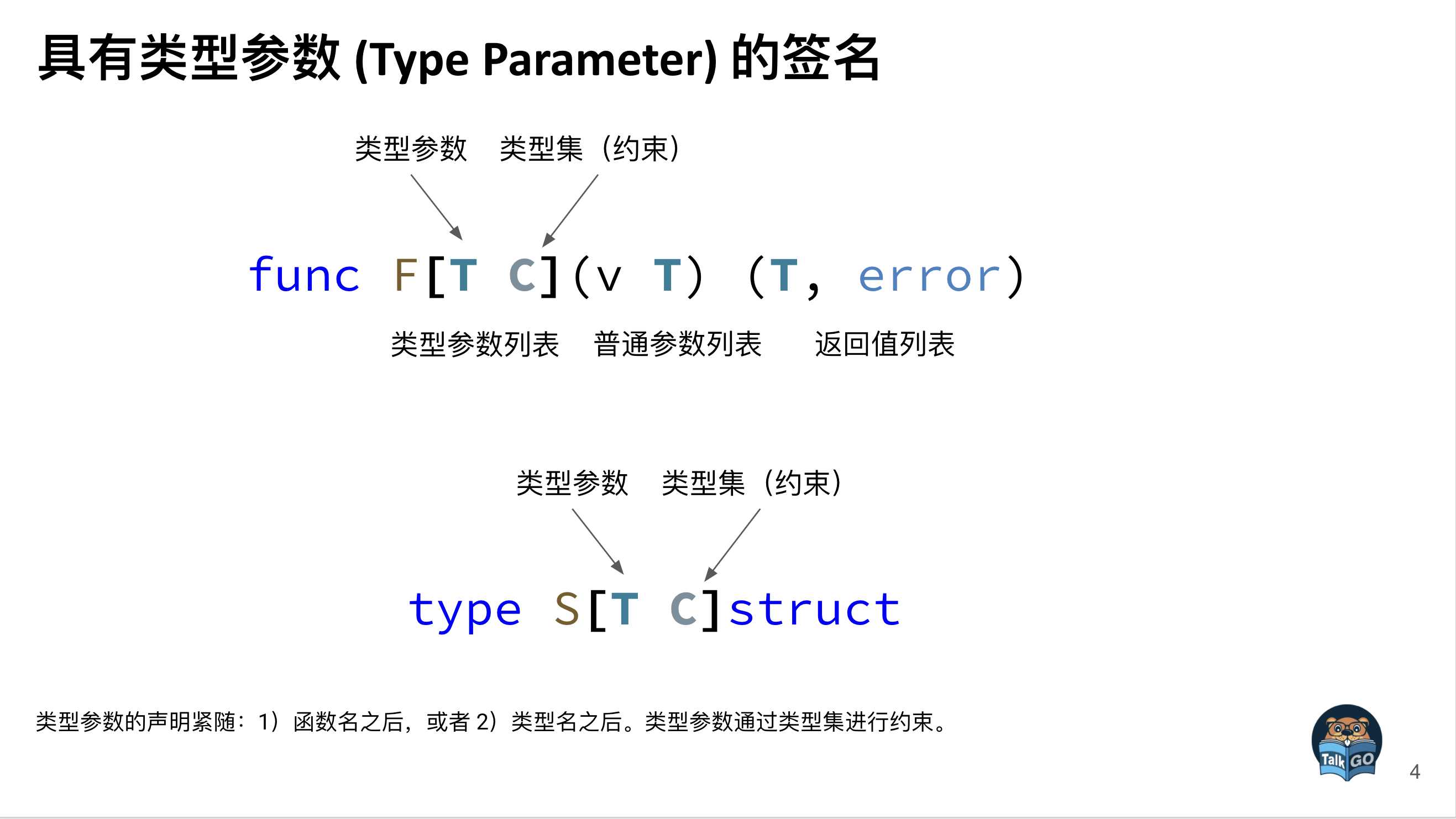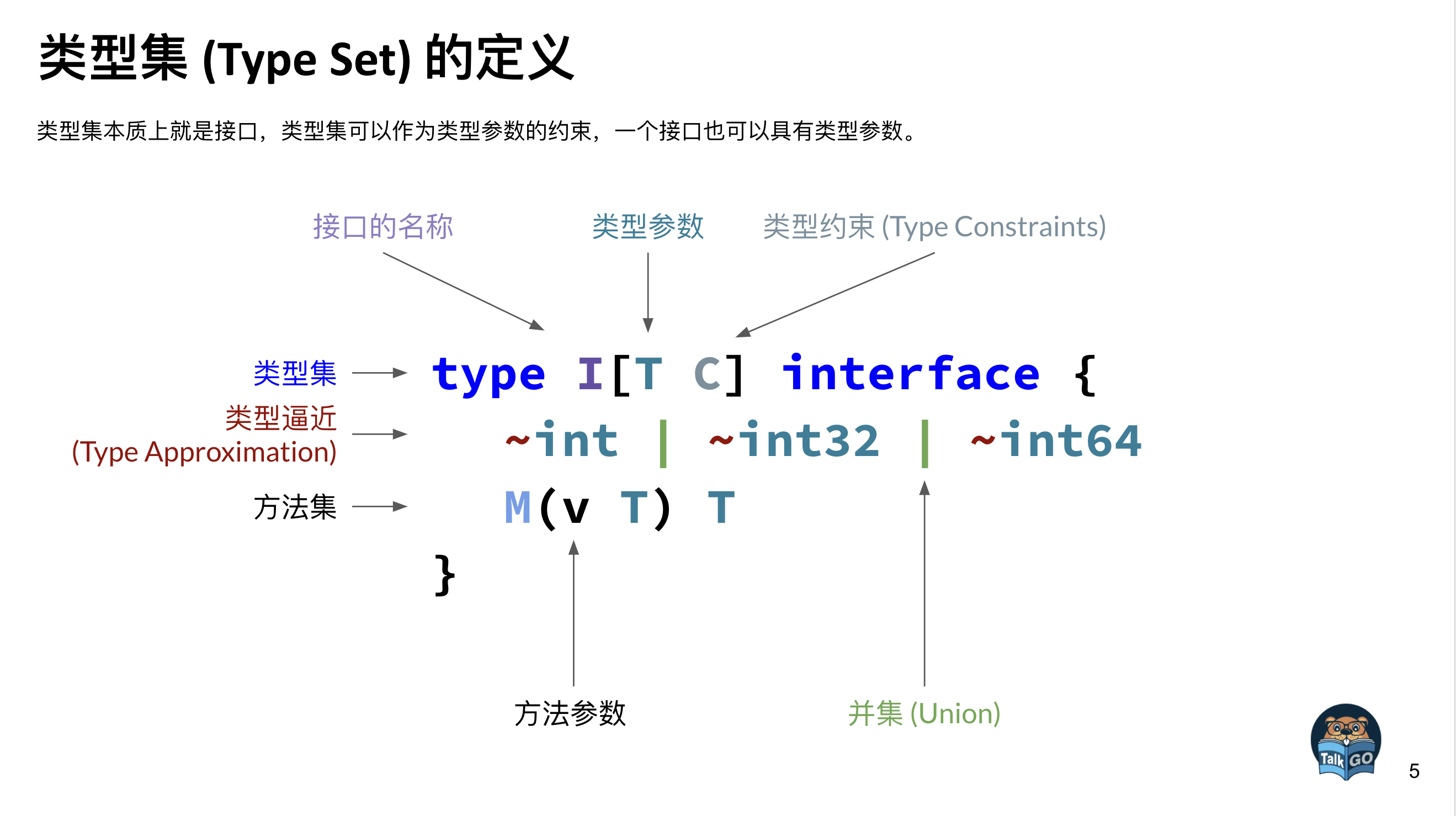# Go1.18泛型试用体验

## 一、 基本使用

### 1.1. 泛式语法简介1. 多了一个类型参数
2. 对类型参数有类型约束，类型约束本质是接口
3. 对于～的理解：～int代表底层类型是int的所有类型，可以是`type MyInt int``type MyIntV2 int`，他们都在～int的范围中

### 1.2. 为什么使用泛式

``````type T interface {
}

func Sum(elems ...T) (sum T) {
if len(elems) == 0 {
return
}
sum = elems
for _, v := range elems[1:] {
}
return
}

// S 类型参数
func GenericSum[S ~int](elems ...S) (sum S) {
if len(elems) == 0 {
return
}
sum = elems
for _, v := range elems[1:] {
sum += v
}
return
}
``````

Sum利用接口实现泛型，GenericSum利用1.18的语言特性，比较两者的区别，我们发现：

1. 当使用接口作为函数的形参类型时，函数调用方传递的实际参数可以是完全不同的类型
2. 当使用类型参数作为函数的形参类型时，函数调用方传递的实际参数必须是满足类型参数所约束的类型

1. 「类型安全的参数传递」：泛型约束并规范了参数类型。

``````type IntSum struct {
Val int
}

func (s *IntSum) Add(t T) T {
s.Val += t.(*IntSum).Val
return s
}

func TestSum(t *testing.T) {
params := make([]T, 0, 4)
params = append(params, &IntSum{Val: 1})
params = append(params, &IntSum{Val: 2})
params = append(params, &IntSum{Val: 3})
params = append(params, &IntSum{Val: 4})

sum := Sum(params...)
fmt.Println(sum.(*IntSum).Val)
}

func TestGenericSum(t *testing.T) {
input := []int{1, 2, 3, 4}

sum := GenericSum[int](input...)

fmt.Println(sum)
}
``````

### 1.3. 什么时候使用泛式

1. 当函数的实现与参数的类型不强相关时，可以考虑使用
2. 实现通用数据结构时，可以考虑
3. 使用性能不应该是使用类型参数的理由，如果使用类型参数能够提高代码的使用场景和阅读的清晰度，则可以考虑使用

1. 如果一个方法的实现对于不同类型都不相同，则不应该考虑使用
2. 类型参数如果既可以用类型参数也可以用纯接口参数，则不应该考虑使用类型参数

### 1.4. 例子

``````type wrapSort[T any] struct {
vals []T
cmp  func(T, T) bool
}

func (s wrapSort[T]) Len() int {
return len(s.vals)
}

func (s wrapSort[T]) Less(i, j int) bool {
return s.cmp(s.vals[i], s.vals[j])
}

func (s wrapSort[T]) Swap(i, j int) {
s.vals[i], s.vals[j] = s.vals[j], s.vals[i]
}

func Sort[T any](s []T, cmp func(T, T) bool) {
sort.Sort(wrapSort[T]{s, cmp})
}
``````

``````type C[T any] interface {
*T
Foo()
Bar()
}

type A struct {
}

func (a A) Foo() {

}

func (a A) Bar() {

}

type B struct {
}

func (b *B) Foo() {

}

func (b B) Bar() {

}

// Want 使用V约束U
func Want[U any, V C[U]]() (x V) {
return
}

func TestWant(t *testing.T) {
a := Want[A]()
b := Want[B]()
fmt.Printf("%T %T\n", a, b)// *A *B
}
``````

### 1.5. 注意点

``````type Ia[T any] interface{ *T }
type Ib[T any] interface{ Foo() }

func bar(T Ia[int]) {} // ERROR: interface contains type constraints
func bar(T Ib[int]) {} // OK
``````

## 二、 泛型标准库

### 2.1. 标准库的变化

``````// golang.org/x/exp/constraints

// Ordered is a constraint that permits any ordered type: any type
// that supports the operators < <= >= >.
// If future releases of Go add new ordered types,
// this constraint will be modified to include them.
type Ordered interface {
Integer | Float | ~string
}
``````

Ordered代表任何可比较的类型

### 2.2. 核心类型

``````type U interface {
*int
String() string
}

type V interface { ~float32 }
type W interface { int | float64 }

// U 的核心类型是 *int，V 的核心类型是 float32，W 没有核心类型．
``````

### 2.3. 运算符

``````func Index[E comparable](s []E, v E) int {
for i, vs := range s {
if v == vs {
return i
}
}
return -1
}

type A struct {
val  int
val1 int
}

func TestIndex(t *testing.T) {
a := A{1, 2}
b := A{2, 3}
c := A{2, 1}
idx := Index[A]([]A{a, b}, c)
fmt.Println(idx)// -1
}
``````

``````func IsSorted[E constraints.Ordered](x []E) bool {
for i := len(x) - 1; i > 0; i-- {
if x[i] < x[i-1] {
return false
}
}
return true
}
``````

## 四、 总结

1. 感觉语法复杂了很多，就是有种你得看两眼才能看明白的代码的感觉；
2. 整体限制好像还比较大，不是很成熟；
3. 做一些无关类型的操作，泛型还是提供了很大的帮助的。

## 五、 遇到的问题

1. mac m1 pro记得下载arm64的（刚下载的时候直接看错了下成amd64的，一直没办法debug，丢人🤦‍♂️）
2. Goland 2021.3.4好像不支持泛型的len操作，会出现报错，但实际编译运行却可以
3. 为什么Go不能用运算符方法？也不是很清楚`comparable和constraints.Ordered`什么场合才会起到限制作用

## 六、 参考资料

1. [#128 Go 1.18 中的泛型【Go 夜读】](